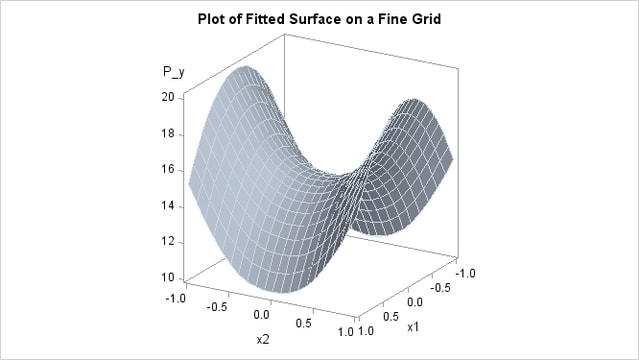## Example 92.1 Partial Spline Model Fit

This example analyzes the data set Measure that was introduced in the section Getting Started: TPSPLINE Procedure. That analysis determined that the final estimated surface can be represented by a quadratic function for one or both of the independent variables. This example illustrates how you can use PROC TPSPLINE to fit a partial spline model. The data set Measure is fit by using the following model: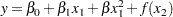The model has a parametric component (associated with the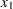variable) and a nonparametric component (associated with the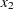variable). The following statements fit a partial spline model:

```data Measure;
set Measure;
x1sq = x1*x1;
run;

data pred;
do x1=-1 to 1 by 0.1;
do x2=-1 to 1 by 0.1;
x1sq = x1*x1;
output;
end;
end;
run;

proc tpspline data= measure;
model y = x1 x1sq (x2);
score data = pred out  = predy;
run;
```

Output 92.1.1 displays the results from these statements.

Output 92.1.1 Output from PROC TPSPLINE
 Raw Data

The TPSPLINE Procedure
Dependent Variable: y

Summary of Input Data Set
Number of Non-Missing Observations 50
Number of Missing Observations 0
Unique Smoothing Design Points 5

Summary of Final Model
Number of Regression Variables 2
Number of Smoothing Variables 1
Order of Derivative in the Penalty 2
Dimension of Polynomial Space 4

Summary Statistics of Final Estimation
log10(n*Lambda) -2.2374
Smoothing Penalty 205.3461
Residual SS 8.5821
Tr(I-A) 43.1534
Model DF 6.8466
Standard Deviation 0.4460
GCV 0.2304

As displayed in Output 92.1.1, there are five unique design points for the smoothing variableand two regression variables in the model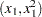. The dimension of the polynomial space is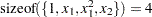. The standard deviation of the estimate is much larger than the one based on the model with bothandas smoothing variables (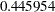compared to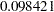). One of the many possible explanations might be that the number of unique design points of the smoothing variable is too small to warrant an accurate estimate for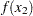.

The following statements produce a surface plot for the partial spline model by using the surface template that is defined in the section Getting Started: TPSPLINE Procedure.

```ods graphics on;

proc sgrender data=predy template=surface;
dynamic _X='x1' _Y='x2' _Z='P_y' _T='Plot of Fitted Surface on a Fine Grid';
run;

ods graphics off;
```

The surface displayed in Output 92.1.2 is similar to the one estimated by using the full nonparametric model (displayed in Output 92.2 and Output 92.6).

Output 92.1.2 Plot of PROC TPSPLINE Fit from the Partial Spline Model